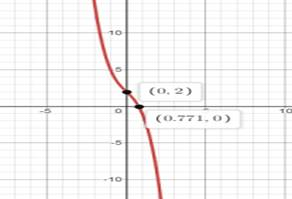# Thevertical and horizontal asymptote of the function.### Single Variable Calculus: Concepts...

4th Edition
James Stewart
Publisher: Cengage Learning
ISBN: 9781337687805### Single Variable Calculus: Concepts...

4th Edition
James Stewart
Publisher: Cengage Learning
ISBN: 9781337687805

#### Solutions

Chapter 4, Problem 7RE

(a)

To determine

## To find: Thevertical and horizontal asymptote of the function.

Expert Solution

There is no Horizontal and vertical asymptote in the function.

### Explanation of Solution

Given: f(x)=2-2x-x3

Concept used:

If the degree of the numerator is more than the denominator, there is no horizontal asymptote.

If the denominator has no zeroes then there has no vertical asymptotes

Or

To get Vertical asymptote function should be rational and denominator must contain some variable otherwise there has no vertical asymptote.

Calculation:

Accordingto the laws of asymptotes:

If the degree of the numerator is more than the denominator, there is no horizontal asymptote.

If the denominator has no zeroes then there has no vertical asymptotes.

Here degree of the numerator is 3 and degree of denominator is 0 .

Hence, there is no Horizontal and vertical asymptote in the function.

(b)

To determine

### To find: TheInterval of increasing or decreasing of the function.

Expert Solution

The function is not real or imaginary so it doesn’t have any zeroes.

The function is neither increasing nor decreasing.

### Explanation of Solution

Given: f(x)=2-2x-x3

Concept used:

Increasing or decreasing function can be calculated by equating first derivative of the function to 0.

dydx=0 .

Zeroes of x can be calculatedafter that the increasing and decreasing can be measured.

Calculation:

Increasing or decreasing function can be calculated by equating first derivative of the function to 0.

dydx=0 .

dydx=-2-3x2

dydx=-2-3x2=0

x2=23

x=±23i

Since the function is not real or imaginary so it doesn’t have any zeroes.

Hence the function is neither increasing nor decreasing.

(c)

To determine

### To find: The Local maxima and minima of the function.

Expert Solution

There is no minima and maxima since the function come up with imaginary.

### Explanation of Solution

Given: f(x)=2-2x-x3 .

Concept used:

The local maxima and minima can be calculated by firstly equating the double differentiation to 0.

1. d2ydx2=0.

2.If d2ydx2>0. the concave will be open upward and local minima can be found.

3. d2ydx2<0. the concave will open downward and local maxima can be found.

Calculation:

f(x)=2-2x-x3

dydx=-2-3x2

dydx=-2-3x2=0

x2=23

x=±23i

d2ydx2=-6x

d2ydx2=-623i

Hence, there is no minima and maxima since the function come up with imaginary.

(d)

To determine

### To find: The interval of concavity and the inflection point.

Expert Solution

x=0 is the point of inflection

Interval of concavity is from (,0), (0,) .

### Explanation of Solution

Given: f(x)=2-2x-x3

Concept used:

The second derivative of function is calculated first.

Set the second derivative equal to zero and solve.

Check whether the second derivative undefined for any values of x.

Plot the number on number line and test the regions with the second derivative.

Plug these 3 values for obtain three inflection points.

The graph of y=f(x) is concave upward on those intervals where

y=f(x)>0 .

The graph of y=f(x) id concave downward on those intervals where

y=f(x)<0 .

If the graph of y=f(x) has point of inflection then y=f(x)=0 .

Calculation:

d2ydx2=-6x =0

x=0 .

Hence, x=0,y=2 is the point of inflection

Interval of concavity is from (,0), (0,)

(e)

To determine

### To Sketch:the graph using graphing use the information from part a to d.

Expert Solution

it’s verified from the graph x=0,y=2 is the point of inflection

Interval of concavity is from (,0), (0,) .

### Explanation of Solution

Given: f(x)=2-2x-x3

Concept used:

The graph can be generated from graphing device called Desmos graphing calculator.

Calculation:

The graph of the function f(x)=2-2x-x3Hence, it’s verified from the graph x=0,y=2 is the point of inflection

Interval of concavity is from (,0), (0,) .

### Have a homework question?

Subscribe to bartleby learn! Ask subject matter experts 30 homework questions each month. Plus, you’ll have access to millions of step-by-step textbook answers!